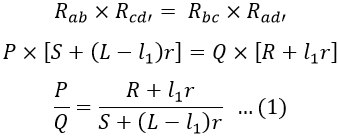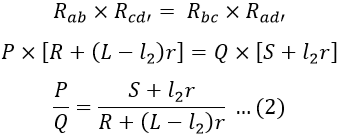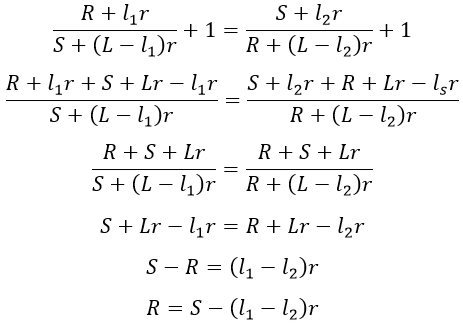# Carey Foster Bridge - Circuit, Working, Applications & Advantages

Carey Foster's bridge is an electrical circuit used to measure the medium value of resistance or resistances which are nearly equal in their value. The construction of Carey Foster's bridge circuit is similar to the Wheatstone bridge which consists of four arms in which four resistances are connected. Carey Foster's bridge is a modification made on the Wheatstone bridge and it works on the same principle i.e., null deflection.

## Carey Foster Bridge Construction & Principle :

Carey Foster slide wire bridge is a modified form of Wheatstone bridge in which a slide wire is introduced in between the standard and unknown resistances. The movable contact i.e., a slider is connected to the galvanometer as shown in the figure below.

The resistances P and Q are chosen such that the ratio of P and Q approximately equals the ratio of R and S i.e., P/R ≃ R/S. The resulting small unbalance in the bridge is corrected by adjusting the position of the slider.

#### In the above figure,P, Q = Known reistancesS = Standard resistanceR = Unknown resistance.

At exact balance, let the slider position be at a distance of l1 cm from the unknown resistance (R) end of the slide wire. The resistances R and S are interchanged and again the slider position is adjusted to a distance l2, where exact balance is achieved.

In order to eliminate errors (or effects) due to thermoelectric EMFs, the reversing switch is thrown from P1 P1' to P2 P2' position (which reverses the supply terminals in the circuit) and the above procedure is repeated for both cases. Finally, the values of l1 and l2 are obtained by taking the average of values achieved in both the positions of reversing switch.

#### Let the resistance per unit length of slide wire be r Ω/cm. At first balance,Similarly, at second balance,From equation 1 and 2, we get,Adding 1 on both sides, we get,Hence, the unknown resistance R can be found in terms of standard resistance, the resistance per unit length of slide wire, and the difference between both slider positions.

If the current is passed for a long time, there will be chances of shifting the balance point, due to a change in resistance of the bridge. The resistance P and Q must be equal, otherwise, it increases the percentage error in the measurement proportionally to the difference between them.

### Applications of Carey Foster's Bridge :

#### The circuit can be used for measuring unknown resistance lying between 1Ω to 100kΩ.It can be used for the measurement or comparison of nearly equal resistances.Used to measure resistance offered per unit length (specific resistance) of the slide wire.To measure the resistance of cells.

Do not enter any spam links and messages